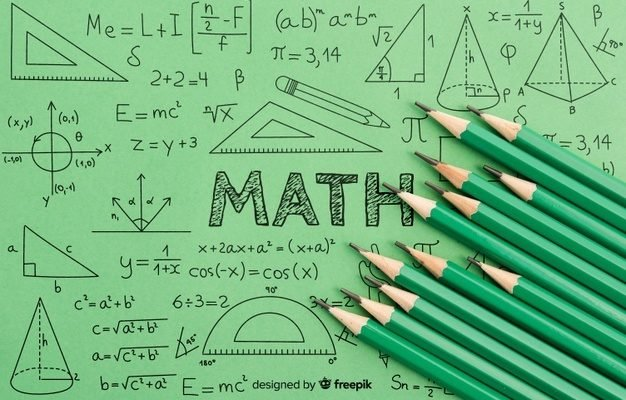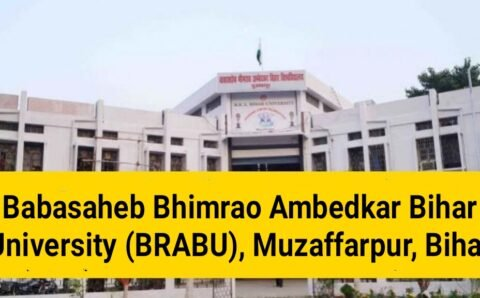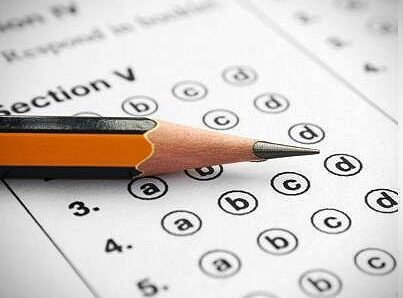# BIHAR UNIVERSITY (Muzaffarpur)MATHEMATICS Exam Important QuestionsBihar university Mathematics Exam Important Questions
(DAY 6)
13-03-2021
Graduation TDC Part 1 (2019-2022) Math/Physics/Chemistry

Exam Important Questions
Graduation TDC Part 1 (2019-2022) Bihar University MATHEMATICS

PG 1st semester (2019-21) – Physics/Math – Important
questions

Are you searching for BSc 1st year and PG 1st semester important questions in Math/Physics/Chemistry ?

Well, you are in the right place. In this post, we are go to share some of the most important questions of Math/Physics/Chemistry (SCIENCE) for TDC Part 1 and PG 1st semester students. (B.R.A BIHAR UNIVERSITY)

## TDC PART 1 MATH(Hons.)

(Day 6)

TDC PART 1 MATH (Hons.)
Paper-1
TDC Part 1 (2019-22)

Group A

## (Answer any six questions, selecting at least one from each Group.)

1. (a) Show that if AcB then (A x B) n (Bx A) = AX A. (b) If A, B, C, be any three non-empty sets then prove that A x (B x)= (A x B) n (A x C)

2. (a) Prove that the set of all real numbers is uncountable.

(b) Prove that the set of algebraic numbers is countable,

3. (a) Prove that the union of countable family of sets is countable.

(b) Prove that the set of rational numbers is denumerable.

4. Prove that ACB and CCD AXC CBXD.

Define partial order relation and total order relation. Give an example of partial order relation which is not a total order relation.

5. (a) Define equivalence class. Prove that two equivalence classes are either disjoint or identical.

(b) If R be an equivalence relation defined in a set A then R is also an equivalence relation.

## Group B

1. (a) Prove that the set of all convex combinations of a finite number of linearly independent vectors, is a convex set.

(b) Prove that the intersection of two convex sets, is also a convex set,

2. (a) Prove that A (adj A)=1 A1.1 = (adj A) A where A is a square matrix.

(b) Solve by graphical method:
Minimize Z = 2x, + 3×2 Subject to the constraints X + x2 21

10x + x 25 * + 10x, 21

3 (a) Show that the collection of all feasible solutions of a l.p. problem is a

Convex set

(b) Show that the sphere is a convex set.

4 (a) If A and B are two non-singular matrices of same order, then show that
(AB)-1 = B- A-1. (b) Define Hermitian and skew Hermitian matrix and show that every square matrix is uniquely expressible as the sum of a Hermitian and a skew Hermitian matrix.

5. (a) Solve the following linear programming problem by graphical method:

Max, Z= 5×1 + 7×2
Subject to be following constraints

X, + x, S4

3x + 8×2 24

10x, + 7×2 s 35

X1, X2 2 0.

## Group C

1. (a) Find the equation whose roots are the roots of x + 4×3 – 2 + 11 = 0 Each diminished by 2

(b) If a, B, y are the roots of the equation x3 + px? + qx+r = 0 find the value of Ea?B.

2. (a) If sin ( + 16) = x+ iy, prove that x2 y2 sin20 cos20 = 1. (b) Show that every equation of nth degree has n roots and no more.

3. (a) Find the condition that the roots a, B, Y, 8 of the equation x + px + qx2 + x + 5 = 0 should be connected by the relation AB =y8.

(b) Using De Moivre’s theorem find the expansion of sin a in ascending powers of a

4. (a) Express sin- (x + iy) in the form A +iB.

(b) Solve x + 4x + 5×2 + 2x 2 = 0 when one of the roots is 1 + 1

5. (a) In an equation with real coefficients, imaginary roots occur in

Conjugate pairs-prove it.
(b) Solve the equation 6×3 + 11×2 – 19x + 6 =0 having given that one of its roots is double the other.

Rn college Telegram group – Click here### स्नातक पार्ट-वन की परीक्षा के लिए क्वेश्चन बैंक बनाने में छूट रहे पसीने, हरेक विभाग को बनाना है 900 सवाल

बीआरए बिहार विश्वविद्यालय में स्नातक पार्ट-वन की परीक्षा के लिए क्वेश्चन बैंक तैयार करने में पीजी विभागों के पसीने छूट रहे हैं। हर विभाग को...### स्नातक पार्ट वन के परीक्षार्थियों को अगले माह मिलेगा मॉडल क्वेश्चन बैंक, परीक्षा के लिए मिल जाएगी अनुमति### BIHAR UNIVERSITY (Muzaffarpur)Zoology Exam Important Questions